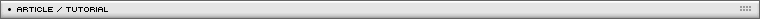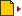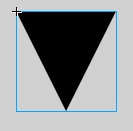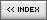Rotating a 3D pyramid
[ July 13, 2004 ] by Eric Lin
Basic 3D in Flash: 3/5
In this third article, the author rotates a three dimensional pyramid.

The first step in rotating a pyramid is defining 4 points 3d data in the format of {x:??,y:??,z:??}. I also calculate the 5th point data, that is the "center" of the pyramid. Later, I'll modify all these data by substracting the 3d vector of the center, so the pyramid center is at (0,0,0) of the 3d space. Well, I dont want to discuss how to set 3d data of these points. You can search the web to define them yourself. If you understand the math, and you go mad, you can make a "3d diamond" with 16 or 36 faces.
The setting up of these 3d data is in frame 1 of the script. To understand how I construct these points might be more difficult than making them by yourself. I implement 3d perspective effect in this pyramid. So, we need to incorporate z data into x,y data and show the effect of scaling. This is a second step, but I will not discuss it here.
The pyramid has 4 faces. The third step is trying to cover these 4 faces with triangle movieClips. This is what I am going to discuss.
The fourth step is adding the rotation of these 4 points. This is already discussed in the 3d cube tutorial.

Now we are going to talk about how to skew a triangle movieClip to cover a face defined by 3 points in {x:??,y:??}. If you don't know how to script a skew by function, you need to check this tutorial. There, we skew a square to conform to p0, width corner pW and height corner pH. How about a triangle?

Well, if our triangle movieClip is a diagonal cut of a square, that function can be applied easily without any need for change. But, pictures will be weird. So, my triangle is something like below. The apex is at the middle of the lower border. That makes pictures look more "normal".How to make the apex of the triangle  to conform to specified point x0,y0? Essentially, this triangle movieClip is in fact a "square" movieClip. When the square skewed, the upper border must be parallel to lower border and with the same length. So, if x0,y0 is the apex of the skewed triangle, then I can calculate the left-bottom corner point x1,y1 by math. If I skew the square by p0,pW, and (x1,y1), then the apex will fall into x0,y0.

Here is the function to get the point that we need to skew our square movieClip. pH is the point apex should be. The return value is the new pH that will be used in skew function.

```function getPH(pt0,pH,pW)
{
var pt=new Object();
pt.x=pH.x+(pt0.x-pW.x)/2;
pt.y=pH.y+(pt0.y-pW.y)/2;
return pt;
}

skewObj(clip, 100, 100, rp0, getPH(rp0,rpH,rpW), rpW);```

Here is the final result: